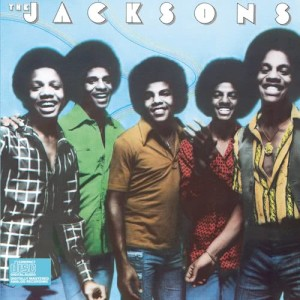Show You the Way to Go

The Jacksons1 Jan 1976

Show You the Way to Go Lyrics

Show You The Way To Go - The Jacksons

Written by：Leon Huff/Kenneth Gamble

Let me show you

Let me show you the way to go

I don't know everything

But there's something I do know

(I know I know)

I've read and heard a lot

And now I'm ready to show

That we can come together

And think like one

Come together now

Live together underneath the sun

Let me show you

Let me show you the way to go

Let me show you come on

Let me show you the way to go

Just put your trust in me

I'll try not to let you down

(Let you down let you down)

The job is hard to do

But only we can work this out

'Cause we can help each other to overcome

We can do it

We can do it now

Rejoice everybody

And get the job done

Just let me show you

Let me show you

Let me show you the way to go

Follow me my friend hey hey

Let me show you

Let me show you the way to go

That we can help each other to overcome

We can do it

We can do it now

Live together underneath the sun

Just let me show you

Let me show you

Let me show you the way to go

I swear I'll never let you down

(Let me show you)

Come on

(Let me show you the way to go)

We can do it we can do it

We can do it now

(Let me show you)

(Let me show you the way to go)

Let me let me let me

Just let me let me show you now

(Let me show you)

Come on

(Let me show you the way to go)

(Let me show you)

(Let me show you the way to go)

I swear

I'll never let you down

Come on

Come on

Let me let me let me show you

Let me let me let me let me show you

Let me show you

Never let you down

Never let you down

Never let you down

We can do it we can do it

We can work it out

And get on top

Let me show you

Let me show you

Let me show you

Let me let me let me

Let me let me let me show you

Let me show you

Let me show you

Let me show you